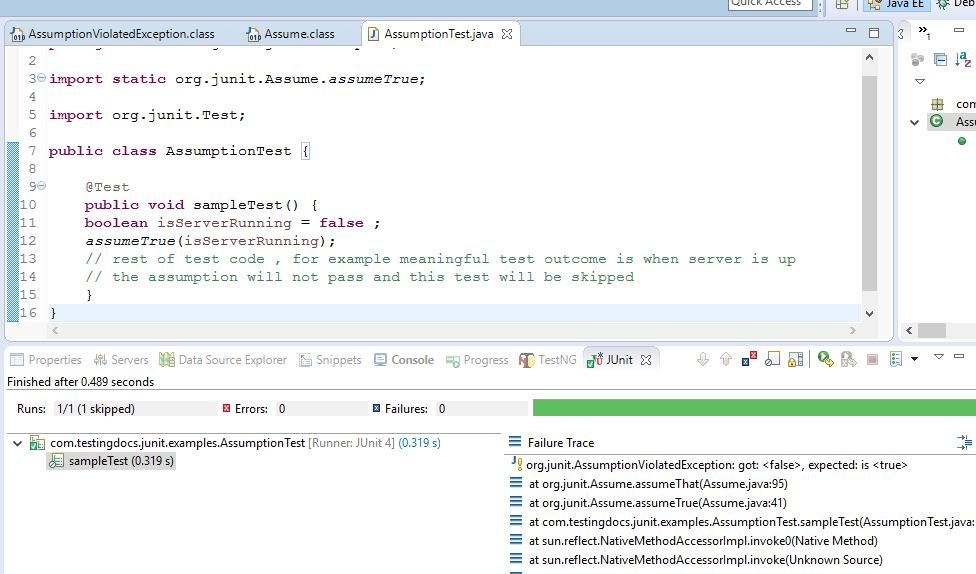# Assumptions in JUnit Tests

Document Contents

### Introduction

In this post, we will discuss assumptions in JUnit tests. Before executing tests we can check out assumptions about the test using the Assume and its methods. If all the assumptions pass then the test is executed for pass /fail. Furthermore, if the assumptions fail then the test is ignored.

Assume class has a set of methods useful for assuming conditions in a test. We can make meaningful assumptions before running the test.

It is important to keep this in mind that: a failed assumption does not mean the code is broken. It means that the test provides no useful information when the assumptions fail. Assume basically means that don’t run the test if the conditions don’t pass. The default JUnit runner skips tests with a failing assume method. Custom runners may behave differently.

### Imports

We can have referenced them through static import as shown below:

import static org.junit.Assume.*;

### Sample Test with Assumptions

```import org.junit.Test;

public class AssumptionTest {

@Test
public void sampleTest() {
boolean isServerRunning = false ;
assumeTrue(isServerRunning);
// rest of test code , for example meaningful test outcome is when server is up
// the assumption will not pass and this test will be skipped
}
}

```

### Screenshot

``

AssumptionViolatedException:

A test for which an assumption fails would throw this exception as shown in the above screenshot.